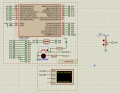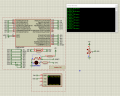# Level sensor simulate

#### crutschow

Joined Mar 14, 2008
27,943
So the maximum sensor output is just 40mV?

So connect a voltage source of 40mV to SN-1 in your picture.
That will give 0 to 40mV at the AD0 output for the potentiometer going from 0% to 100%.

•Camiloo

#### Camiloo

Joined Aug 21, 2021
8
So the maximum sensor output is just 40mV?

So connect a voltage source of 40mV to SN-1 in your picture.
That will give 0 to 40mV at the AD0 output for the potentiometer going from 0% to 100%.
Okay, I already did. I am using an ATMega328P, how do I make the relationship in the programming so that 2.5 meters correspond to 10mV? I tried to make the relationship but I get 10mV / 2m

#### ericgibbs

Joined Jan 29, 2010
13,861
hi Cam,

E

•Camiloo

#### Camiloo

Joined Aug 21, 2021
8
hi Cam,

E
Hey, I tried with this.

Code:
#define SN1 A0
#define SN2 A1

float vRef = 0;
int nivelSN1;
int voltajeSN1;

void setup()
{
Serial.begin(9600);
}

void loop()
{
vRef = voltaje_Ref();
voltajeSN1 = Escalizar(nivelSN1, 0, 8, 0, 40);
nivelSN1 = Escalizar(voltajeSN1, 0, 40, 0, 10);
Serial.print(voltajeSN1); Serial.println(nivelSN1);
delay(500);
}

float Escalizar(float variable, float in_min, float in_max, float out_min, float out_max)
{
return (variable - in_min) * (out_max - out_min) / (in_max - in_min) + out_min;
}
And proteus...This is a point of a project, so I need this for doing the rest of conditions..#### ericgibbs

Joined Jan 29, 2010
13,861
hi C,
If you are using the internal 5V ref for the ADC, which give 1023 counts for 5V input, but for 0.04V
input to the ADC that will only be 1023(0.04/5) = 8 Counts, much to coarse to resolve to 2.5mtr.

E
You should add a OPA amplifier from the pot to the ADC input.

•Camiloo

#### Camiloo

Joined Aug 21, 2021
8

#### ericgibbs

Joined Jan 29, 2010
13,861
I am not using the 5V reference,
Hi,
So what ADC reference voltage value are you using.?
E

#### Camiloo

Joined Aug 21, 2021
8
hi C,
If you are using the internal 5V ref for the ADC, which give 1023 counts for 5V input, but for 0.04V
input to the ADC that will only be 1023(0.04/5) = 8 Counts, much to coarse to resolve to 2.5mtr.

E
You should add a OPA amplifier from the pot to the ADC input.
Okay, I will try adding a amplifier, but, should I use 5V or 40mV input to the potentiometer?

#### Camiloo

Joined Aug 21, 2021
8

#### Camiloo

Joined Aug 21, 2021
8
hi,
I would use the internal 5Vref and supply the pot with 5V.
[the same 5V as the Arduino]
In the sketch use the MAP command.

E

example.
Change the 255 to 100, then some maths to make it 10.0 mtrs
View attachment 246194
Thanks for the help, I did it this way, will it be okay? I don't use the map command because I need the 2.5 meters in the output, if I use map it throws me as an integer type and I only get a 2.Code:
#define SN1 A0
#define SN2 A1

float vRef = 0;
float nivelSN1;
float voltajeSN1;

void setup()
{
Serial.begin(9600);
}

void loop()
{
vRef = voltaje_Ref();
voltajeSN1 = Escalizar(nivelSN1, 0, 1023, 0, (vRef*8));
nivelSN1 = Escalizar(voltajeSN1, 0, (vRef*8), 0, 10);

Serial.print("V: "); Serial.print(voltajeSN1); Serial.println(" mV");
Serial.print("Distance: "); Serial.print(nivelSN1); Serial.println(" meters");
delay(500);
}

float Escalizar(float variable, float in_min, float in_max, float out_min, float out_max)
{
return (variable - in_min) * (out_max - out_min) / (in_max - in_min) + out_min;
}

float voltaje_Ref()
{
long result;
float respuesta;
ADMUX = _BV(REFS0) | _BV(MUX3) | _BV(MUX2) | _BV(MUX1);
delay(2);
result = (1125300L / result);
respuesta = result / 1000.0;
return respuesta;
}

Last edited:

#### ericgibbs

Joined Jan 29, 2010
13,861
hi C,
The result you have posted looks OK.
Are you able to read from 0m thru 10.0mtrs in the 2.5mtr steps OK.
E

BTW:
For future reference you could MAP the 1023 count to 1000 and use maths to give 0.0m the 10.0mtr

•Camiloo

#### Camiloo

Joined Aug 21, 2021
8
hi C,
The result you have posted looks OK.
Are you able to read from 0m thru 10.0mtrs in the 2.5mtr steps OK.
E

BTW:
For future reference you could MAP the 1023 count to 1000 and use maths to give 0.0m the 10.0mtr
Thanks a lot! you are the best <3

•Delta Prime

#### ericgibbs

Joined Jan 29, 2010
13,861
hi Camiloo.
For reference,this sketch is the way I was suggesting, simpler than your coding.

E

C-like:
// Water Height Camiloo 21-08-2021

float Water= 0;

void setup() {
Serial.begin(9600);
}

void loop() {

Water = map(ADC0in, 0, 1023, 0, 100); // Map 1023 to 100 units
Water= ((Water/10)); // divide by 10 for decimal

Serial.print("Water= ");
Serial.print(Water,1); //print to One decimal place
Serial.println("mtr");
delay(1000);
}